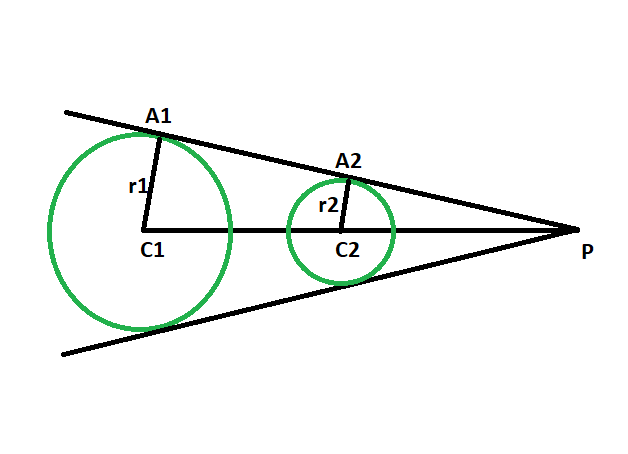# Ratio of the distance between the centers of the circles and the point of intersection of two direct common tangents to the circles

Given two circles of given radii, such that the circles don’t touch each other, the task is to find the ratio of the distance between the centers of the circles and the point of intersection of two direct common tangents to the circles.

Examples:

```Input: r1 = 4, r2 = 6
Output: 2:3

Input: r1 = 22, r2 = 43
Output: 22:43
```Approach:

• Let the radii of the circles be r1 & r2 and the centres be C1 & C2 respectively.
• Let P be the point of intersection of two direct common tangents to the circles, and A1 & A2 be the point of contact of the tangents with the circles.
• In triangle PC1A1 & triangle PC2A2,
angle C1A1P = angle C2A2P = 90 deg { line joining the center of the circle to the point of contact makes an angle of 90 degree with the tangent },
also, angle A1PC1 = angle A2PC2
so, angle A1C1P = angle A2C2P
as angles are same, triangles PC1A1 & PC2A2 are similiar.
• So, due to similiarity of the triangles,
C1P/C2P = C1A1/C2A2 = r1/r2

The ratio of the distance between the centres of the circles and the point of intersection of two direct common tangents to the circles = radius of the first circle : radius of the second circle

Below is the implementation of the above approach:

## C++

 `// C++ program to find the ratio of the distance ` `// between the centers of the circles ` `// and the point of intersection of ` `// two direct common tangents to the circles ` `// which do not touch each other ` ` `  `#include ` `using` `namespace` `std; ` ` `  `// Function to find the GCD ` `int` `GCD(``int` `a, ``int` `b) ` `{ ` `    ``return` `(b != 0 ? GCD(b, a % b) : a); ` `} ` ` `  `// Function to find the ratio ` `void` `ratiotang(``int` `r1, ``int` `r2) ` `{ ` `    ``cout << ``"The ratio is "` `         ``<< r1 / GCD(r1, r2) ` `         ``<< ``" : "` `         ``<< r2 / GCD(r1, r2) ` `         ``<< endl; ` `} ` ` `  `// Driver code ` `int` `main() ` `{ ` `    ``int` `r1 = 4, r2 = 6; ` `    ``ratiotang(r1, r2); ` `    ``return` `0; ` `} `

## Java

 `// Java program to find the ratio of the distance ` `// between the centers of the circles ` `// and the point of intersection of ` `// two direct common tangents to the circles ` `// which do not touch each other ` `class` `GFG { ` ` `  `    ``// Function to find the GCD ` `    ``static` `int` `GCD(``int` `a, ``int` `b) ` `    ``{ ` `        ``return` `(b != ``0` `? GCD(b, a % b) : a); ` `    ``} ` ` `  `    ``// Function to find the ratio ` `    ``static` `void` `ratiotang(``int` `r1, ``int` `r2) ` `    ``{ ` `        ``System.out.println(``"The ratio is "` `                           ``+ r1 / GCD(r1, r2) ` `                           ``+ ``" : "` `                           ``+ r2 / GCD(r1, r2)); ` `    ``} ` ` `  `    ``// Driver code ` `    ``public` `static` `void` `main(String args[]) ` `    ``{ ` `        ``int` `r1 = ``4``, r2 = ``6``; ` `        ``ratiotang(r1, r2); ` `    ``} ` `} ` ` `  `// This code has been contributed by 29AjayKumar `

## Python3

 `# Python 3 program to find the ratio  ` `# of the distance between the centers  ` `# of the circles and the point of intersection  ` `# of two direct common tangents to the circles ` `# which do not touch each other ` ` `  `# Function to find the GCD ` `from` `math ``import` `gcd ` ` `  `# Function to find the ratio ` `def` `ratiotang(r1, r2): ` `    ``print``(``"The ratio is"``, ``int``(r1 ``/` `gcd(r1, r2)), ``":"``,  ` `                          ``int``(r2 ``/` `gcd(r1, r2))) ` ` `  `# Driver code ` `if` `__name__ ``=``=` `'__main__'``: ` `    ``r1 ``=` `4` `    ``r2 ``=` `6` `    ``ratiotang(r1, r2) ` ` `  `# This code is contributed by ` `# Surendra_Gangwar `

## C#

 `// C# program to find the ratio of the distance ` `// between the centers of the circles ` `// and the point of intersection of ` `// two direct common tangents to the circles ` `// which do not touch each other  ` `using` `System; ` `     `  `class` `GFG ` `{ ` ` `  `    ``// Function to find the GCD ` `    ``static` `int` `GCD(``int` `a, ``int` `b) ` `    ``{ ` `        ``return` `(b != 0 ? GCD(b, a % b) : a); ` `    ``} ` ` `  `    ``// Function to find the ratio ` `    ``static` `void` `ratiotang(``int` `r1, ``int` `r2) ` `    ``{ ` `        ``Console.WriteLine(``"The ratio is "` `                        ``+ r1 / GCD(r1, r2) ` `                        ``+ ``" : "` `                        ``+ r2 / GCD(r1, r2)); ` `    ``} ` ` `  `    ``// Driver code ` `    ``public` `static` `void` `Main(String[] args) ` `    ``{ ` `        ``int` `r1 = 4, r2 = 6; ` `        ``ratiotang(r1, r2); ` `    ``} ` `} ` ` `  `// This code contributed by Rajput-Ji `

## PHP

 ` `

Output:

```The ratio is 2 : 3
```

My Personal Notes arrow_drop_upCheck out this Author's contributed articles.

If you like GeeksforGeeks and would like to contribute, you can also write an article using contribute.geeksforgeeks.org or mail your article to contribute@geeksforgeeks.org. See your article appearing on the GeeksforGeeks main page and help other Geeks.

Please Improve this article if you find anything incorrect by clicking on the "Improve Article" button below.

Article Tags :
Practice Tags :

Be the First to upvote.

Please write to us at contribute@geeksforgeeks.org to report any issue with the above content.